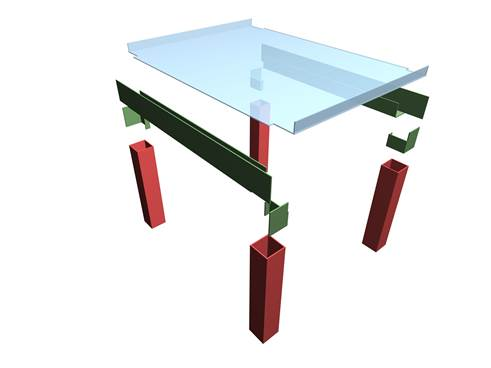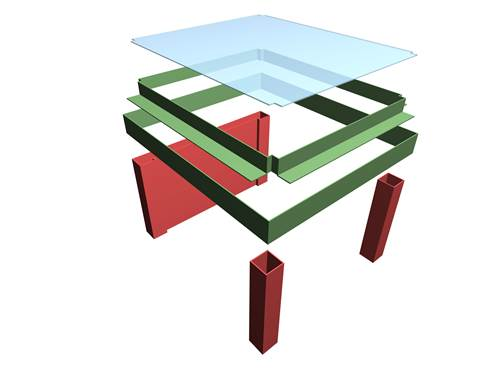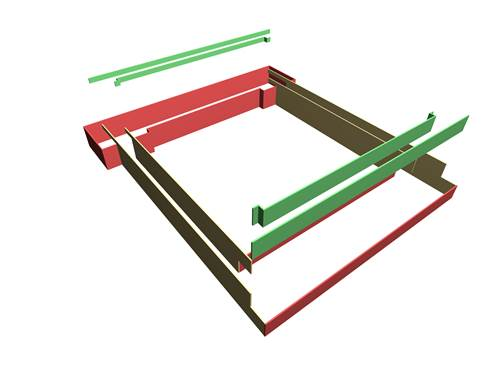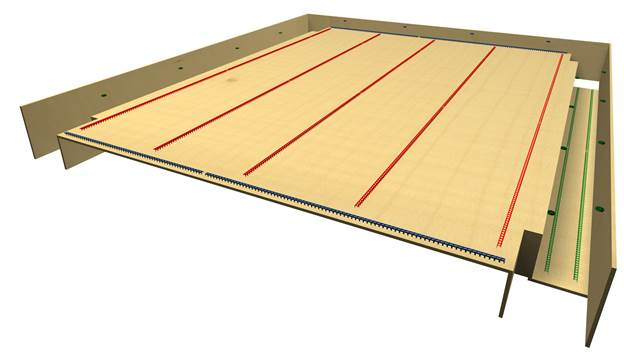# Formwork quantity take-offThe structural frame
The construction
The reinforcement I
The reinforcement II
Quantity/Cost estimation
Detailing drawings
Introduction >Wind and Seismic Forces >
Structural model and Analysis
Slabs
Seismic behavour of frames
Appendix A
Appendix B
Appendix C
Appendix D
Introduction >
Modelling slabsMaterials
To be continued >
Introduction

## Formwork quantity take-off of ground floorBeams – Slabs m2 S1: 3,80 x 5,00 – 4 x 0,40 x 0,10 + 2 x 3,60 x 0,18 = 20,136 b1,b2: 2 x 5,00 x 0,50 + 2 x (5,00-2 x 0,40) x 0,32 + + 2 x 4,20 x 0.30 = 10,208 Columns (joints areas): 4 x 0,40 x 0,50 + 4 x 0,40 x 0,32 + 4 x 0,10 x 0,32 = 1,440 Total = 31,784 Columns: 4 x (0,40 x 0,40 x 2,50) = 16,000

## Formwork quantity take-off of basementBeams – Slabs m2 S1: 4,50 x 3,90 - 4 x (0,15 x 0,15) = 17,460 b1,b2: 2 x [5,00 x 0,50 +4,20 x 0,32] +4,20 x 0,25 = 9,788 b3: 4,40 x 0,50 + 3,60 x 0,32 +3,60 x 0,25 = 4,252 Shear wall sw5: 4,40 x 0,50 + 3,60 x 0,32 = 3,352 internal column sides: 4 x 2 x 0,15 x 0,32) = 0,384 Total = 35,236 Columns: 4 x (0,40 x 0,40 x 2,50) – 2 x (0,25 x 2,50) = 14,750 Shear wall sw5: 2 x (3,60 x 2,50) = 18,000 Total = 32,750

## Formwork quantity take-off of foundationm2 Footings F3,4,5: 0,50 x [5,00 + 2 x 1,00 + 0,30 x 2] = 5,85 Cb1,2: 2 x [0,70 x0,10 + 4,30 x 0,60 +0,30 x 0,30] 2 x [0,30 x0,10 + 3,60 x 0,60 +0,30 x 0,30] = 10,04 Sf4: (3,80 + 4,40) x 0,30 + (3,60 + 4,40) x 0,30 = 4,86 Columns: 2 x 2 [0,10 x 0,30 +0,10 x 0,10] + +(4,40+3,60 ) x 0,10 = 0,96 Total = 21,71

## Spacers quantity take-offRULES FOR ESTIMATING THE QUANTITTIES OF SPACERS AND REBAR CHAIRS
Superstructure and foundation beams:       (2 m of ‘linear spacers’ + 2 ‘pieces of point support’) in every meter of ‘clear beam length’
Columns:       (8 ‘pieces of point support’) per column
Shear walls:       2 m of ‘linear spacers’ in every meter of the shear wall’s length
Foundation slabs and footings:      (1.25 m of ‘linear spacers’) in every square meter of ‘clear slab area’
Slabs’ free edges:       (1 m of ‘linear spacers’ + 1m of ‘rebar chairs’ + 1 ‘piece of point support’) in every edge meter
Slabs’ supports:       1 m of ‘rebar chairs’ per meter of ‘every slab support’